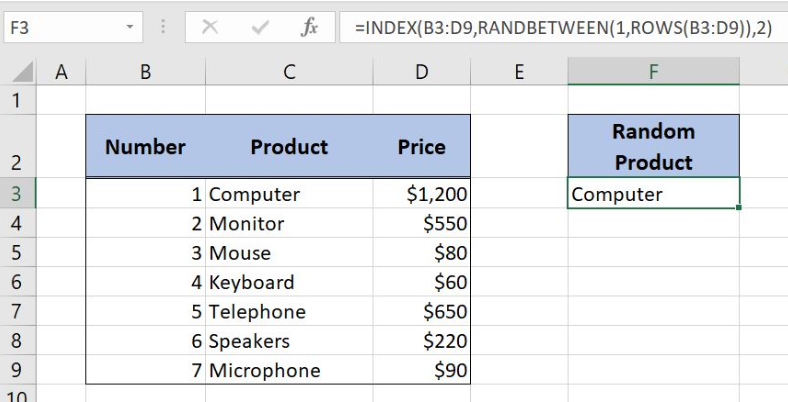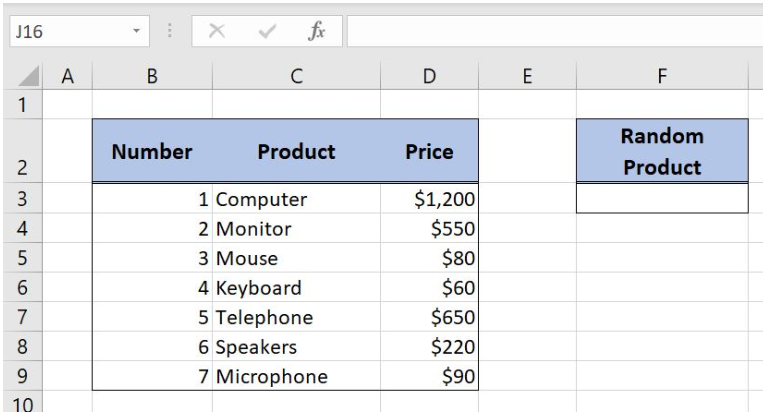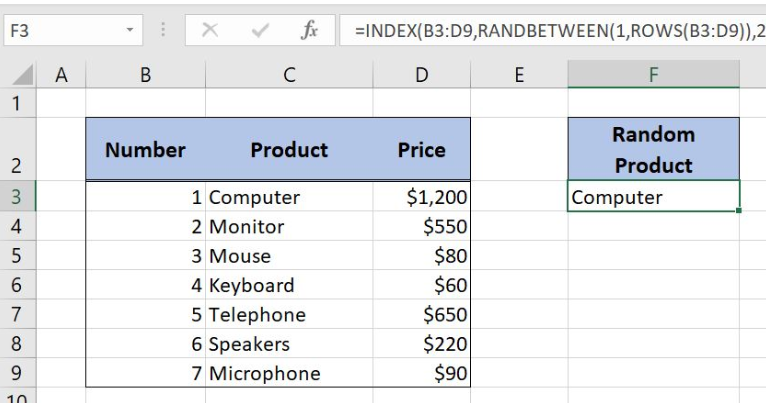Get instant live expert help with Excel or Google Sheets“My Excelchat expert helped me in less than 20 minutes, saving me what would have been 5 hours of work!”

#### Post your problem and you’ll get expert help in seconds.

Your message must be at least 40 characters
Our professional experts are available now. Your privacy is guaranteed.

# Discover How to Generate a Random Number in a Table in Excel

Excel allows a user to generate a random number in a table using the INDEX, RANDBETWEEN and ROWS functions. This step by step tutorial will assist all levels of Excel users to discover how to generate a random number in a table in Excel.Figure 1. The final result of the formula

## Syntax of the INDEX formula

The generic formula for the INDEX function is:

`=INDEX(array, row_num, column_num)`

The parameters of the INDEX function are:

• array – a range of cells where we want to get a data
• row_num – a number of a row in the array for which we want to get a value
• column_num – a column in the array which returns a value.

## Syntax of the RANDBETWEEN Formula

The generic formula for the RANDBETWEEN function is:

`=RANDBETWEEN(bottom, top)`

The parameters of the RANDBETWEEN function are:

• bottom – a value from which we want to get a random value
• top – a value to which we want to get a random value.

## Syntax of the ROWS formula

The generic formula for the ROWS function is:

`=ROWS(array)`

The parameter of the ROWS function is:

• array – a range of cells which rows we want to count.

## Setting up Our Data for the Example

Let’s look at the structure of data that we will use. In the table, we have 3 columns: “Number” (column B), “Product” (column C) and “Price” (column D). In the cell F3, we want to get a random product from the table B3:D9.Figure 2. Data that we will use in the example

## Generate a Random Value from the Table

We want to get a random product from column C of the table in the cell F3.

The formula in F3 looks like:

`=INDEX(B3:D9, RANDBETWEEN(1, ROWS(B3:D9)), 2)`

The parameter array of the ROWS function is the range B3:D9. The result of the ROWS function is the top parameter of the RANDBETWEEN function, while the bottom is 1. This function returns a random row number of a table. This is the row_num parameter of the INDEX function. The array parameter is B3:D9, while the column_num is 2, as we want to get a value from the second column of the table.

To apply the formula, we need to follow these steps:

• Select cell F3 and click on it
• Insert the formula: `=INDEX(B3:D9,RANDBETWEEN(1,ROWS(B3:D9)),2)`
• Press enter.Figure 3. Using the formula to generate a random value from a table

The ROWS function returns 7, as our table has 7 rows. Therefore, the RANDBETWEEN returns a random number between 1 and 7, in our case 1. Finally, the INDEX function returns the value from row 1 and column 2 of the table. The result in the cell F3 is “Computer”.

Most of the time, the problem you will need to solve will be more complex than a simple application of a formula or function. If you want to save hours of research and frustration, try our live Excelchat service! Our Excel Experts are available 24/7 to answer any Excel question you may have. We guarantee a connection within 30 seconds and a customized solution within 20 minutes.

Solution examplesI need help on index and match where the return is not correct
Solved by B. B. in 20 minsI have a database extract that is showing the same clients multiple times in the list. How can I write a formula in excel to look at the column with the client names and return only unique records on another sheet? I want to run this over several files so wanting to avoid using pivot tables which is how I would normal do this.
Solved by X. J. in 20 minsI need to create a pivot table that sums visits per day by provider: Date...Visits....Provider So, I can ask...how many visits did provider X have on day Y?
Solved by F. J. in 34 minscould someone breakdown this formula for me :D =ArrayFormula(IF(D\$5>=ROWS(B\$5:B5),INDEX(Sheet1!C\$6:C\$21,SMALL(IF(Sheet1!D\$6:D\$21=\$E\$5,ROW(Sheet1!C\$6:C\$21)-ROW(Sheet1!C\$6)+1),ROWS(B\$5:B5))),""))
Solved by C. C. in 40 minsHi, I have created a drop down list and in another cell I have used an IF function which is dependent on the drop down list. However, when i change the selected option from the drop down list, the IF function cell does not update accordingly
Solved by C. C. in 17 mins